# Kullback Leibler Divergence

## Definition

Let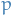and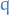be two probability distributions for a random variable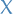.

The Kullback-Leibler (KL) divergence

• ifis discrete, is given by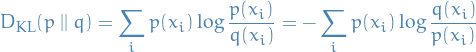• ifis continuous,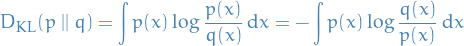## Interpretation

### Probability

Kullback-Leibner divergence from some probability-distributions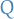and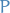, denoted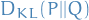, is a measure of the information gained when one revises one's beliefs from the prior distributionto the posterior distribution. In other words, amount of information lost whenis used to approximate.

Most importantly, the KL-divergence can be written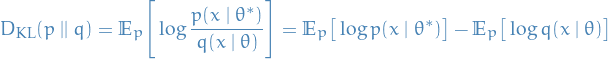where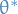is the optimal parameter and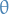is the one we vary to approximate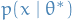. The second term in the equation above is the only one which depend on the "unknown" parameter(is fixed, since this is the parameter we assumeto take on). Now, suppose we have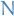samples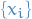from, then observe that the negative log-likelihood for some parametrizable distributionis given by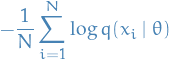By the Law of Large numbers, we have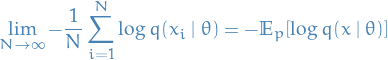where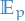denotes the expectation over the probability density. But this is exactly the second term in the KL-divergence! Hence, minimizing the KL-divergence betweenand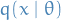is equivalent of minimizing the negative log-likeliood, or equivalently, maximizing the likelihood!

### Coding

The Kraft-McMillan theorem establishes than any decodable coding scheme for coding a message to identify one value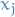out of a set of possibilitiescan be seen as representing an implicit probability distribution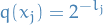over, where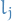is the length of the code forin bits

Therefore, we can interpret the Kullback-Leibner divergence as the expected extra message-length per datum that must be commincated if a code that is optimal for a given (wrong) distributionis, compared to using a code based on the true distribution.

Let's break this a bit down:

• Kraft-McMillan theorem basically says when taking the exponential of the length of each valid "codeword", the resulting set (of exponentials) looks like a probability mass function.
• If we were to create a coding schema for the values in(our set of symbols) using binary representation (bits) using our "suggested" probability distribution, KL-divergence gives us the expected extra message-length ber datum compared to using a code based on the true distribution